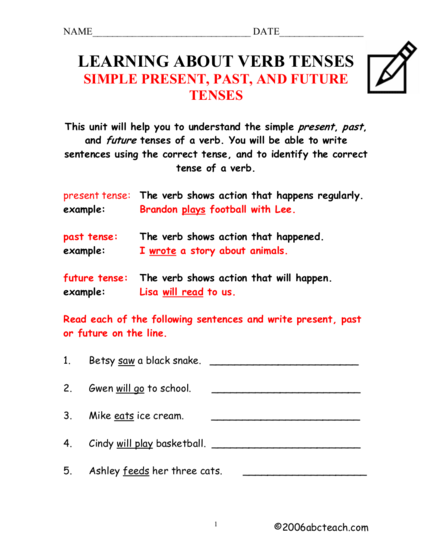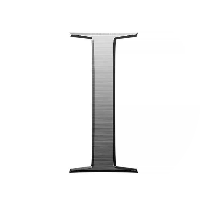# Verb Tenses: Simple Present, Past and Future TensesContributed by:This presentation will teach the most basic form of tenses in all three settings. The most predominant tense is a simple tense. Simple tenses are the basic versions of past, present, and future tense verbs.

1. SIMPLE PRESENT, PAST, AND FUTURE
a ele ee
ptm ic re
ee T wrote a story about animals.
future tenses
ne ea
Read each of the following sentences and write present, past
or future on the line.
; re
2. SIMPLE PRESENT, PAST, AND FUTURE
Lo aaa ie
Read each of the following sentences. Circle the correct tense
Cn nn |
ee a a
ee a a
ee a a
ee a a
ee a a
3. SIMPLE PRESENT, PAST, AND FUTURE
Lr aera Er
Choose the correct verb to match the tense in the (parentheses)
ee re
ee ee re
ee re
ee ee ee
ee re
ee re
a re
ee ee ee
ee re
ee re
: re
4. SIMPLE PRESENT, PAST, AND FUTURE
PRACTICE 3
Write a sentence using an action verb in the correct tense.

5. SIMPLE PRESENT, PAST, AND FUTURE
PRACTICE 4
eR eR eye Reel Rue
tense verbs, and make an x under the future tense verbs.
a ae ee
a ee a
an a a
ee a an
ae a an
a ee ee
a a a
a ae a
ae a ee
: re
6. ae ee
7 a
7 ee
ee a
ee re
ee a ae a a a
a a a an
a ee a
a ee
i re
7 re